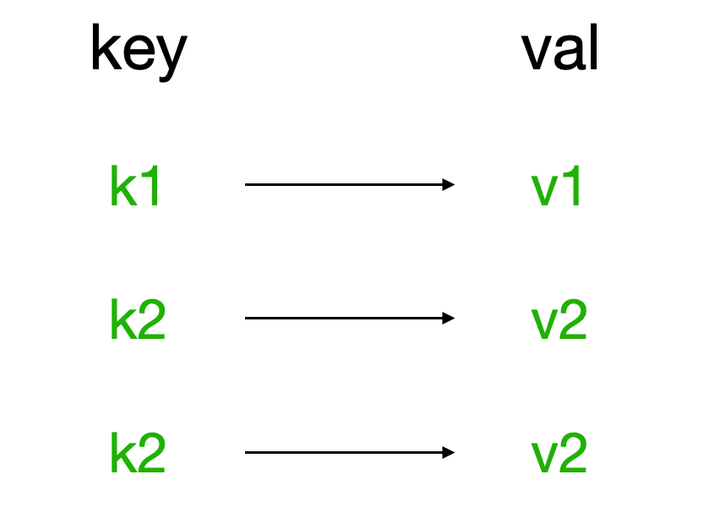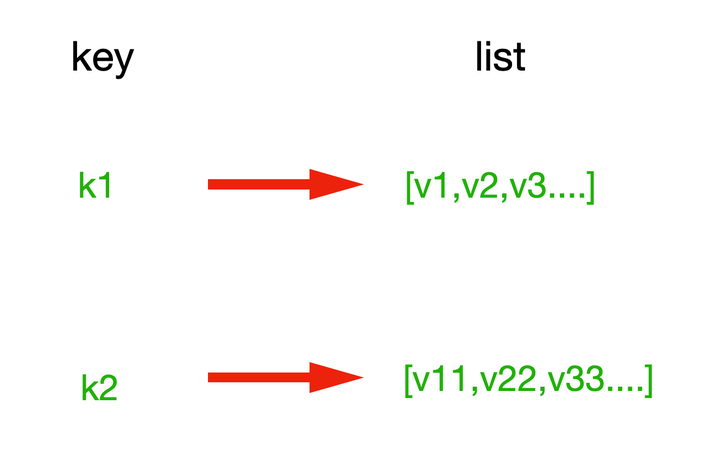# Python 分布式缓存之Reids数据类型操作详解

10/18 12:49

1、Redis API

1.安装redis模块

``\$ pip3.8install redis``

2.使用redis模块

``import redis # 连接redis的ip地址/主机名，port，password=Noner = redis.Redis(host="127.0.0.1",port=6379,password="gs123456")``

3.redis连接池

redis-py使用connection pool来管理对一个redis server的所有连接，避免每次建立、释放连接的开销。默认，每个Redis实例都会维护一个自己的连接池。可以直接建立一个连接池，然后作为参数Redis，这样就可以实现多个Redis实例共享一个连接池

``import redis# 创建连接池，将连接保存在连接池中pool = redis.ConnectionPool(host="127.0.0.1",port=6379,password="gs123456",max_connections=10) # 创建一个redis实例，并使用连接池"pool"r = redis.Redis(connection_pool=pool)``

2、String 操作

redis中的String在内存中按照一个name对应一个value来存储。如图：1. set 为name设置值

``# 在Redis中设置值，默认，不存在则创建，存在则修改set(name, value, ex=None, px=None, nx=False, xx=False, keepttl=False) name：设置键value：设置值ex：设置过期时间（秒级）px：设置过期时间（毫秒）nx：如果设置为True，则只有name不存在时，当前set操作才执行,同setnx(name, value)xx：如果设置为True，则只有name存在时，当前set操作才执行``

set用法：

``r.set("name1","jack",ex=3600)r.set("name2","xander",xx=36000)``

setnx用法：

``# 设置值，只有name不存在时，执行设置操作（添加）setnx(name, value)``

setex用法：

``# 设置值,参数：time -->过期时间（数字秒 或 timedelta对象）setex(name, value, time)``

psetex用法：

``# 设置值,参数：time_ms，过期时间（数字毫秒 或 timedelta对象）psetex(name, time_ms, value)``

2. get 获取name的值

``# 根据key获取值get(name) r.get("foo")``

3. mset 批量设置name的值：

``mset(mapping) data = { "k1":"v1", "k2":"v2",}r.mset(data)``

4. Mget 批量获取name的值

``# 批量获取值，根据多key获取多个值mgets(mapping) # 方法一r.mget("k1","k2") # 方法二data = ["k1","k2"]r.mget(data) # 方法三data = ("k1","k2")r.mget(data)``

5. getset 设置新值并获取原来的值

``getset(name, value) r.set("foo", "xoo")ret = r.getset("foo", "yoo")print(ret) # b'xoo'``

6. append 为name原有值后追加内容

``# key对应值的后面追加内容append(key, value) r.set("name","jack")r.append("name","-m")ret = r.get("name")print(ret) # b'jack-m'``

7. strlen 返回name的值字节长度：

``# 返回字符串的长度，当name不存在时返回0strlen(name) r.set("name","jack-")ret = r.strlen("name")print(ret) # 5``

8. incr 为name整数累加值

``# 自增mount对应的值，当mount不存在时，则创建mount＝amount，否则，则自增,amount为自增数(整数)incr(name, amount=1) r.incr('mount')r.incr('mount')r.incr('mount', amount=3)ret = r.get('mount')print(ret)      # b'5'``

3、Hash 操作

hash表现形式上有些像pyhton中的dict,可以存储一组关联性较强的数据 ，redis中Hash在内存中的存储格式如下图：1. hset 为name设置单个键值对

``# name对应的hash中设置一个键值对（不存在，则创建；否则，修改）hset(name, key, value) name：设置namekey：name对应hash中的key（键）value：name对应的hash中的value（值）``

hset用法

``# 一次只能设置一个键值对r.hset("student-jack", "name", "Jack")``

2 . hget 获取name单个键值对

``# 根据name对应的hash中获取根据key获取valuehget(name,key) ret = r.hget("student-jack", "name")print(ret) // b'Jack'``

3. hmset 为name设置多个键值对

``# mapping中传入字典（不存在，则创建；否则，修改)hmset(name, mapping):  data = { "name": "Jack", "age": 20, "gender": "M",}r.hmset("student-jack", mapping=data)``

4. hmget 获取name多个键值对

``# 根据name对应的hash中获取多个key的值hmget(name, keys, *args) name：指定namekeys：要获取key集合，如：['k1', 'k2', 'k3']*args：要获取的key，如：k1,k2,k3  # 直接传入需要获取的键ret = r.hmget("student-jack", "name", "age")print(ret) # [b'Jack', b'20'] # 列表中指定需要获取的键data = ["name", "age"]ret = r.hmget("student-jack", data)print(ret) # [b'Jack', b'20']``

5. hgetall 获取name的键值对

``# 根据name获取hash的所有值hgetall(name) ret = r.hgetall("student-jack")print(ret)  # {b'name': b'Jack', b'age': b'20', b'gender': b'M'}``

6、hlen 获取name中的键值对个数

``# 根据name获取hash中键值对的总个数hlen(name) ret = r.hlen("student-jack")print(ret) # 3 ， 3个键值对``

7. hkeys 获取name中键值对所有key

``# 获取name里键值对的keyhkeys(name) ret = r.hkeys('student-jack')print(ret) # [b'name', b'age', b'gender']``

8. hvals 获取name中键值对所有value

``# 获取name里键值对的valuehvals(name) ret = r.hvals('student-jack')print(ret) # [b'Jack', b'20', b'M']``

9. hkeys 检查name里的键值对是否有对应的key

``# 根据name检查对应的hash是否存在当前传入的keyhexists(name, key) # 返回布尔值ret = r.hexists('student-jack', 'name')print(ret)  # True``

10. hincrby 从name里的键值对设置自增值

1.整数自增：

``# 自增name对应的hash中的指定key的值，不存在则创建key=amounthincrby(name, key, amount=1) name：设置键key：hash对应的keyamount：自增数（整数）  ret = r.hincrby('student-jack', 'age')ret = r.hincrby('student-jack', 'age')print(ret)      # 22``

2.浮点自增

``# 自增name对应的hash中的指定key的值，不存在则创建key=amounthincrbyfloat(name, key, amount=1.0) name：设置键key：hash对应的keyamount：自增数（浮点数）``

11. hdel 根据name从键值对中删除指定key

``# 根据name将对应hash中指定的key键值对删除hdel(name,*keys)  r.hdel("info",*("m-k1","m-k2"))``

4、List 操作

List操作，redis中的List在内存中按照一个name对应一个List来存储。如图：1. lpush 为name添加元素，每个新的元素都添加到列表的最左边

``# name对应的list中添加元素lpush(name,values)  # 直接指定多个元素r.lpush("names", "Jack", "Alex", "Eric") # 将需要添加的元素添加到元组data = ("Jack", "Alex", "Eric")r.rpush("names", *data) # 将需要添加的元素添加到列表data = ["Jack", "Alex", "Eric"]r.rpush("names", *data)``

Note：列表类型中的值统称元素

2. rpush 为name添加元素，每个新的元素都添加到列表的最右边

``# 同lpush，但每个新的元素都会添加到列表的最右边rpush(name, values)``

3. lpushx 为name添加元素，只有当name已存在时，将元素添加至列表最左边

``lpushx(name,value)``

4. rpushx 同上，将元素添加至列表最右边

``rpushx(name, values)``

5. llen 统计name中list的元素个数

``# name对应的list元素的个数llen(name) ret = r.llen('names')print(ret) # 3， 该list中有3个元素``

6. linsert 为name中list的某一个值或后 插入一个新的值

``# 在name对应的列表的某一个值前或后插入一个新值linsert(name, where, refvalue, value) name：设置namewhere：BEFORE或AFTERrefvalue：标杆值，即：在它前后插入数据value：要插入的数据  // 在Alex值前插入一个值（BEFORE表示：在...之前）r.linsert('names', 'BEFORE', 'Jack', 'Jason') // 在Jack后插入一个值（AFTER表示：在...之后）r.linsert('names', 'AFTER', 'Jack', 'Xander')``

7. lset 为name中list的某一个索引位置的元素重新赋值

``# 对name对应的list中的某一个索引位置重新赋值lset(name, index, value) name：设置nameindex：list的索引位置value：要设置的值 // 将索引为1的元素修改为Gigir.lset('names', 1, 'Gigi')``

8. lrem 移除name里对应list的元素

``# 在name对应的list中删除指定的值lrem(name, count, value) name：设置namevalue：要删除的值count：count=0，删除列表中的指定值； count=2,从前到后，删除2个； count=-2,从后向前，删除2个 r.lrem('names', count=2, value='Xander')``

9. lpop 从name里的list获取最左侧的第一个元素，并在列表中移除，返回值是则是第一个元素

``lpop(name) ret = r.lpop('names')print(ret)      # b'Jason'``

10. rpop 同上，从右侧获取第一个元素

``rpop(name)``

11. lindex 在name对应的列表 根据索引获取元素

``# 在name对应的列表中根据索引获取列表元素lindex(name, index) ret = r.lindex('names', 0)print(ret)  # b'Gigi'``

12. ltrim 移除列表内没有在该索引之内的值（截断）

``# 移除列表内没有在该索引之内的值ltrim(name, start, end) r.ltrim("names",0,2)``

13. lrange 在name对应的列表 根据索引获取数据

``# 在name对应的列表分片获取数据lrange(name, start, end) name：设置namestart：索引的起始位置end：索引结束位置 // 先添加点元素data = ['Jack', 'Eric', 'Koko', 'Jason', 'Alie']r.rpush('names', *data) // 获取列表所有元素ret = r.lrange('names', 0, -1)print(ret) # [b'Gigi', b'Alex', b'Jack', b'Eric', b'Koko', b'Jason', b'Alie'] // 获取列表索引2-5的元素（包含2和5，即 2 3 4 5）ret = r.lrange('names', 2, 5)print(ret)  # [b'Jack', b'Eric', b'Koko', b'Jason'] // 获取列表的最后一个元素ret = r.lrange('names', -1, -1)print(ret)  # [b'Alie']``

0
0 收藏

### 作者的其它热门文章0 评论
0 收藏
0